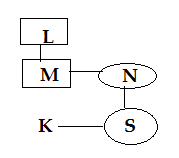# SSC CGL 2018 Practice Test Papers | Reasoning Ability (Day-58)

Dear Aspirants, Here we have given the Important SSC CGL Exam 2018 Practice Test Papers. Candidates those who are preparing for SSC CGL 2018 can practice these questions to get more confidence to Crack SSC CGL 2018 Examination.

[WpProQuiz 3713]

Click “Start Quiz” to attend these Questions and view Explanation

1) In the following question, select the related word from the given alternatives.
Tom : Molly : : ? : ?

a) Cock: Hen

b) Cow : Calf

c) Doe: Fawn

d) Vixen : Cub

2) In the following question, select the related number from the given alternatives.

16 : 254 : : 18 : ?

a) 320

b) 324

c) 326

d) 322

3) In the following question, select the odd letters from the given alternatives.

a) AZ

b) IS

c) MN

d) GT

4) Arrange the given words in the sequence in which they occur in the dictionary.

i) Gesture

ii) Guess

iii) Genuine

iv) General

a) i. iv, iii, ii

b) iii. ii. iv. i

c) iv, iii, i, ii

d) i, iii, ii, iv

5) In the following question, select the missing number from the given series.

4, 27, 73, 142, ?, 349

a) 254

b) 267

c) 298

d) 234

6) In the following question, select the word which cannot be formed using the letters of the given word.

QUALIFICATION

a) LIAR

b) LION

c) FICTION

d) FLAT

7) If “P” denotes “divided by”, “R” denotes “added to”, “S” denotes “subtracted from” and “Q” denotes “multiplied by”, then

48 P 4 R 3 Q 4 S 6 Q 4 =?

a) 1

b) 2

c) 3

d) 0

8) In the following question, select the number which can be placed at the sign of question mark (?) from the given alternatives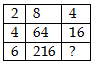a) 42

b) 64

c) 36

d) 72

9) A is taller than B but Shorter C. C is taller than both D and B. Who can be  the shortest one?

a) A

b) B

c) D

d) Cannot be determined

10) – If ‘A + B’ means ‘A is father of B’, ‘A – B’ means ‘A is mother of B’, ‘A × B’ means ‘A is brother of B’ and ‘A÷B’ means ‘A is sister of B’, then how is M related to S in ‘L + M × N – S ÷ K’?

a) Paternal Uncle

b) Maternal Uncle

c) Brother

d) Brother in law

11) How many rectangles are there in the given figure?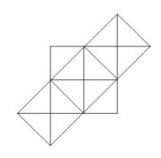a) 14

b) 15

c) 17

d) 18

Molly is female cat; Tom is male cat. Similarly Hen is Female and Cock is male bird

16 = 162 – 2 = 256 – 2 = 254

18 = 182 – 2 = 324 – 2 = 322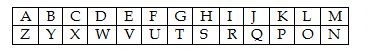The correct order is

iv). General

iii). Genuine

i). Gesture

ii) Guess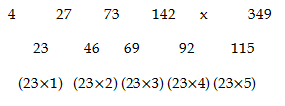48 / 4 R+3 x 4 S-6 Qx4 =0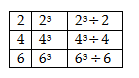Possibility I -CDAB

Possibility III- CABD